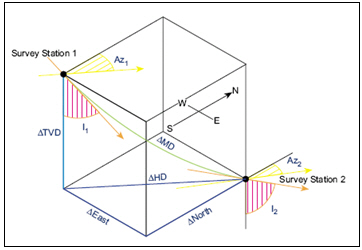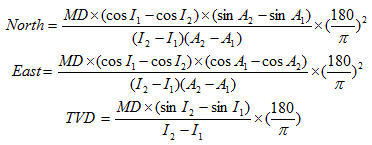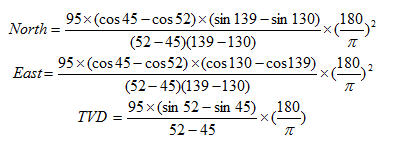Radius of Curvature Method is the most famous method for directional survey calculation. You can calculate North, East and TVD between directional surveys based on the Radius of Curvature Method as per formula below:Where;

MD = measured depth between surveys in ft

I1 = inclination (angle) at upper survey in degrees

I2 = inclination (angle) at lower in degrees

Az1= Azimuth direction at upper survey

Az2 = Azimuth direction at lower survey

Calculation example for Radius of Curvature Method

Survey 1

Depth = 7500 ft

Inclination = 45 degree (I1)

Azimuth = 130degree (A1)

Survey 2

Depth = 7595 ft

Inclination = 52 degree (I2)

Azimuth = 139 degree (A2)

MD = 7595 – 7500 = 95 ftAs per the calculation above,

North = -49.97 ft

East = 50.66 ft

TVD = 62.91 ft

Please find the Excel sheet used for directional survey calculation with Radius of Curvature Method.